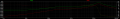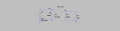# Inductor circuit resonant frequency

#### samudavid

Joined Jan 4, 2018
29
Hello everyone and thank you for helping.

I'm trying to design a coil driver using a transformer. L1 and L2 are coupled and L3 is the load coil. To transfer the maximum power to the load, I should use the resonant frequency of the system. I thought that Fres = 1/(2π*√C2L1) which is about 2.8Khz.
It turns out that the resonant frequency is actually about 16KHz as you can see in the plot attached.

I've tried to do the calculations using the T model, but the resultant inductance (1mH) is far away from expected (66uH for 16KHz).

I don't understand well what is happening. Why L3 affects resonant frequency and how to calculate the equivalent inductance so I can know what the resonant frequency will be in advance.

Any clarification is wellcome.

Thanks again.#### Papabravo

Joined Feb 24, 2006
20,399
You have not decoupled L2 and L3. L1 "sees" the parallel combination of L2 and L3.

#### samudavid

Joined Jan 4, 2018
29
Thanks Papabravo!

However, I don't understand how resonant frequency has to be calculated. I've tried L1+L2//L3 and L1//(L2//L3) and it's not as simulated. The simulation, plus the resonant frequency formula gives a estimated 66uH for 16Khz and 1414nF capacitor.

Any idea?

#### Danko

Joined Nov 22, 2017
1,737
I don't understand how resonant frequency has to be calculated. I've tried L1+L2//L3 and L1//(L2//L3) and it's not as simulated. The simulation, plus the resonant frequency formula gives a estimated 66uH for 16Khz and 1414nF capacitor.
L1, L2 is transformer.
So inductance of L2, L3 in parallel transforms to left part of circuit as 9.68 uH * (L1 / L2) = 67.91 uH.
Transformed 67.91 uH is connected in parallel with 2105 uH (L1).
Therefore total inductance, connected to capacitor C2 is 65.78 uH.

Last edited: